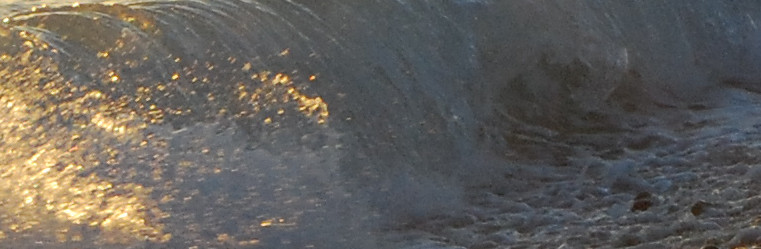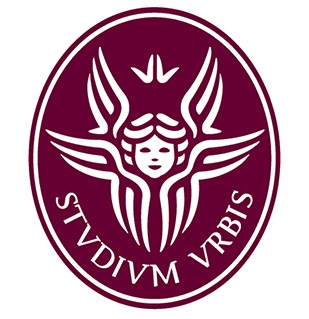Gabriella Puppo Dipartimento di Matematica
La Sapienza Universita' di Roma
Piazza Aldo Moro 5
00185 Roma
ItalyCourses at Sapienza, 2018/2019

• Metodi numerici di approssimazione, Laurea in Matematica: numerical methods for ODE's, theory and applications.
• Istituzioni di Matematica 2, Laurea in Architettura: advanced calculus.
Courses taught to Math and Physics students at the University of Insubria.
• Numerical methods for PDE's B: finite element methods for elliptic and parabolic problems (second semester, 2017/2018, and every other year) Master level.
• Topics in Numerical analysis: approximation of functions, discrete Fourier transform, approximation of integrals, numerical integration of Ordinary Differential Equations (second semester, every year).
• Numerical methods for PDE's A: finite volume methods for hyperbolic problems and balance laws (second semester, 2016/2017, and every other year) Master level.
In all cases, except Istituzioni di Matematica 2, the course consists in theoretical lectures and lab sessions with Matlab, to experiment live the algorithms studied.